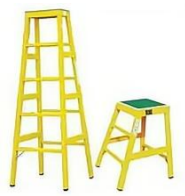$\text{A.}$ 不锈钢 $\text{B.}$ 铁 $\text{C.}$ 铝合金 $\text{D.}$ 木头